Lithologic Analysis of Rock Volume
This page is a highly abbreviated version of Chapter 13 on this website. For more about mineralogy, alternate methods and examples, go to the Main Index Page.

The third step in a log analysis is to calculate the volume of each component of the matrix rock. A large number of minerals exist and you must choose a set of likely minerals appropriate to the rocks present in the zone. The minerals present must be differentiable with sufficient resolution to provide useful results. Minerals with similar properties are often lumped together.

We can solve for the volume of one mineral for each porosity indicating log over the zone. Thus a sonic, density, neutron combination or photoelectric effect, density, neutron combination can provide volumes for either two or three mineral models. YOU must choose the correct two or three minerals for the end points of the model. Choosing the wrong end points may lead to mathematically feasible results which are not correct.

If additional lithology indicating curves are available, for example the natural or induced spectral gamma ray logs, one more mineral may be added to the model for each useable curve. Such models cannot be computed by hand, and computer programs are required. {n some software, porosity can be one of the “minerals”.

When gas is present, only the photo electric effect (two mineral model) is useful for hand calculated lithology analysis. If only one porosity indicating log is present, the lithology will be, by definition, the lithology you imposed on the porosity calculation done earlier.

The META/ESP spreadsheet, available on the Downloads tab at www.spec2000.net, handles these models and makes the work relatively painless.

Lithology calculations from logs are calibrated by comparison to sample descriptions, core descriptions, thin section point counts and X-ray diffraction data.Two Mineral Lithology From Matrix Density

This is the easiest and most common lithology method and is widely used in wellsite and office computer programs.NORMAL CASES:

STEP 1: Calculate shale density from shale porosity (a constant for each zone):

1: DENSSH = PHIDSH * KD1 + (1 – PHIDSH) * KD2

PHIDSH and DENSSH are constants for each zone, chosen from the density log in a nearby shale.

STEP 2: Translate density porosity for each layer to density units:

2: DENS = PHID * KD1 + (1 – PHID) * KD2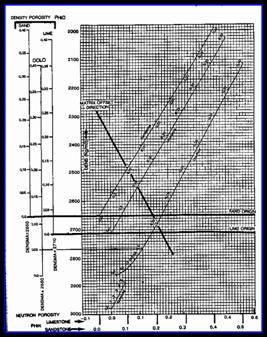Where:
KD1 = 1.00 for English units

KD1 = 1000 for Metric units

KD2 = 2.65 for English units Sandstone scale log

KD2 = 2650 for Metric units Sandstone scale log

KD2 = 2.71 for English units Limestone scale log

KD2 = 2710 for Metric units Limestone scale log

KD2 = 2.87 for English units Dolomite scale log

KD2 = 2870 for Metric units Dolomite scale log

STEP 3: Calculate matrix density:

3: DENSma = (DENS – PHIe * DENSW – Vsh * DENSSH)
/ (1 – PHIe – Vsh)

STEP 4: Calculate rock volumes:

4: Min1 = (DENSma – DENS2) / (DENS1 – DENS2)

5: Min2 = 1.0 – Min1SPECIAL CASES:

Cannot be used in gas zones.

To use this method for sonic neutron crossplot, replace all DENSxx terms in Equations 3, 4, and 5 with their corresponding DTCxx terms. Equations 1 and 2 are not needed.USAGE RULES:

·         If Min1 and Min2 are to be plotted in a volumetric track with Vsh and PHIe, multiply by Vrock before plotting, where Vrock = 1 – PHIe – Vsh.

·         Use any time data is available, but not in bad hole conditions or when gas is present. Methods using PE or UMA are usually better.

·         This equation will break down when PHIe plus Vsh approaches 1.0, so we limit the use of the equation to those cases where PHIe + Vsh < 0.8.Lithology From Sonic Density Neutron Data

This is usually called the M-N method in the literature. This method provides two different two-mineral and one three-mineral models.

YOU must choose the model which gives the best resolution for the mineral end points you have chosen. Resolution is better when the values for the end points have the largest absolute difference.NORMAL CASES:

STEP 1: Shale correct log data:

1: PHIdc = PHID – (Vsh * PHIDSH)

2: PHInc = PHIN – (Vsh * PHINSH)

If PHIN is in sandstone units, subtract 0.03 before using it.

3: PHIsc = (DTC – (1 – Vsh) * 47.3 – (Vsh * DTCSH)) / (KS1 – 47.3)

Note: DTC must be in English units (us/ft).

4: DENSc = PHIdc * KD1 + (1 – PHIdc) * 2.71

5: DTCc = PHIsc * KS1 + (1 – PHIsc) * 47.3Where:
KD1 = 1.0 for fresh drilling mud

KD1 = 1.1 for salty drilling mud

KS1 = 200 for fresh drilling mud

KS1 = 188 for salty drilling mud

STEP 2: Calculate lithology factors:

6: Mlith = 0.01 * (KS1 – DTCc) / (DENSc – KD1)

7: Nlith = (1.00 - PHInc) / (DENSc – KD1)

8: Klith = Mlith / Nlith

9: Alith = 1 / Nlith

STEP 3: Calculate two mineral rock volumes from MLITH factor:

10: Min1 = (Mlith – MLITH2) / (MLITH1 – MLITH2)

11: Min2 = 1.0 – Min1

STEP 4: Calculate two mineral rock volumes from NLITH factor:

12: Min1 = (Nlith – NLITH2) / (NLITH1 – NLITH2)

13: Min2 = 1.0 – Min1

STEP 5: Calculate three mineral rock volumes from Mlith and Nlith:

14: D = (Mlith * (NLITH2 – NLITH1) + Nlith * (MLITH1 – MLITH2)

+ MLITH2 * NLITH1 – MLITH1 * NLITH2) / (MLITH1 * (NLITH3 – NLITH2)

+ MLITH2 * (NLITH1 – NLITH3) + MLITH3 * (NLITH2 – NLITH1))

15: E = (D * (NLITH3 – NLITH1) – Nlith + NLITH1) / (NLITH1 – NLITH2)

16: Min1 = MAX(0, 1 – D – E) / (MAX(0, 1 – D – E) + MAX(0, D) + MAX(0, E))

17: Min2 = MAX(0, E) / (MAX(0, 1 – D – E) + MAX(0, D) + MAX(0, E))

18: Min3 = 1 – Min1 – Min2

SPECIAL CASES:

Cannot be used in gas zones.

To use DTCma and DENSma instead of Mlith and Nlith, replace all Mlith terms with DTCma terms, and all Mlith with DENSma terms in Equations 15 and 16. Equations 1 thru 14 are not needed, but DTCma and DENSma must be derived as shown in Section 6.01.USAGE RULES:

·         If Min1 and Min2 are to be plotted in a volumetric track with Vsh and PHIe, multiply by Vrock before plotting, where Vrock = 1 - PHIe – Vsh. For examp;e Vmin1 = Min1 * Vrock.

·         Nlith gives about the same answer as the matrix density method, because both methods use density and neutron data. Do not use in bad hole conditions or when gas is present.

·         Mlith uses sonic and density data. Do not use in bad hole conditions or when gas is present.

·         Alith and Klith can be used to calculate 2 or 3 mineral models by replacing all Mlith and Nlith terms in Equations 10 through 18 with corresponding Alith and Klith terms.

·         Klith uses sonic and neutron data and can be used in bad hole where Mlith and Nlith are no good.

·         For the available two or three mineral models in this section, choose the method with the most resolution for the mineral pair you have chosen. This means that the numerical distance between the end points is as large as possible.

·         Mlith and Nlith are usually called M and N, but they can be confused with the cementation exponent M and the saturation exponent N, so we have changed their names to reduce confusion.PARAMETERS: See next Section

To calculate MLITH and NLITH parameters for salt mud case, use Equations 6 and 7 with KD1 and KS1 for salt mud.Lithology From PE Density Neutron Data

This is the best method for calculating lithology if the data is available. This method provides two different two-mineral and one three-mineral models.

YOU must choose the model which gives the best resolution for the mineral end points you have chosen. Resolution is better when the values for the end points have the largest absolute difference.NORMAL CASES:

STEP 1: Calculate shale density and shale capture cross section (a constant for each zone):

1: DENSSH = PHIDSH * KD1 + (1 – PHIDSH) * KD2

2: USH = PESH * DENSSH

STEP 2: Translate density porosity to density units for each layer:

3: DENS = PHID * KD1 + (1 –  PHID) * KD2

Where:  KD1 = 1.00

KD2 = 2.65 for Sandstone scale

KD2 = 2.71 for Limestone scale

NOTE: Density data is needed in English units (gm/cc).

STEP 3: Calculate matrix capture cross section for each layer:

4: Uma = (PE * DENS – Vsh * USH) / (1 – PHIe)

STEP 4: Calculate two mineral rock volumes from UMA:

5: Min1 = (Uma – UMA2) / (UMA1 – UMA2)

6: Min2 = 1.0 – Min1

STEP 5: Calculate two mineral rock volumes from PE:

7: Min1 = (PE – PE2 – PESH * Vsh) / (PE1 – PE2)

8: Min2 = 1.0 – Min1

STEP 6: Calculate three mineral rock volumes from Uma and DENSma:

9: D = (Uma * (DENS2 – DENS1) + DENSma * (UMA1 – UMA2)

+ UMA2 * DENS1 – UMA1 * DENS2) / (UMA1 * (DENS3 –  DENS2)

+ UMA2 * (DENS1 – DENS3) + UMA3 * (DENS2 –DENS1))

10: E = (D * (DENS3 – DENS1) – DENSma + DENS1) / (DENS1 – DENS2)

11: Min1 = MAX(0, 1 – D – E) / (MAX(0, 1 – D – E) + MAX(0, D) + MAX(0, E))

12: Min2 = MAX(0, E) / (MAX(0, 1 - D - E) + MAX(0, D) + MAX(0, E))

13: Min3 = 1 – Min1 – Min2SPECIAL CASES:

Only the PE 2 mineral model can be used in gas zones.

To use DTCma instead of Uma, replace all Uma terms with DTCma terms in Equations 9 and 10. Equations 1 thru 8 are not needed, but DTCma and DENSma must be derived as shown in Section 6.01.USAGE RULES:

·         If Min1 and Min2 are to be plotted in a volumetric track with Vsh and PHIe, multiply by Vrock before plotting, where Vrock = 1 - PHIe – Vsh.For example, Vmin1 = Min1 * Vrock.

·         Use the Uma method any time data is available, but not in bad hole conditions or when gas is present.

·         Use the PE method any time data is available, but not in bad hole conditions. However it can be used when gas is present.

·         There may be three mineral combinations where the sonic density neutron methods have better resolution. Check the shape of the mineral triangles.

·         Porosity can also be obtained from the PE response equation. Since resolution is poor, this method is not recommended – the density/PE combination is better.

·         If this method is used to find the fraction of clay minerals present in a shale, Uma is found from Uma = PESH * DENSsh / (1 - BVWSH).  Then the two or three mineral model is applied.

·         If natural gamma ray spectral log data is available, other two and three mineral models can be solved in similar fashion as for PE density neutron.

·         If clay is to be one of the minerals to be found along with two matrix rocks, then do not shale correct the Uma equation, and use PHIt instead of PHIe in Step 2.PARAMETERS:

*                                PHINMA      DENSMA        DTCMA          MLITH     NLITH             PE                   UMA

Clean Quartz              – 0.028              2650                 182        0.802     0.623              1.82                    4.8

Calcite                         0.000                2710                 155        0.822     0.585              5.09                  13.8

Dolomite                     0.005                2870                 144        0.769     0.532              3.13                    9.0

Anhydrite                    0.002                2950                 164        0.707     0.512              5.08                  15.0

Gypsum                      0.507                2350                 172        1.002     0.365              4.04                    9.5

Mica Muscovite           0.165                2830                 155        0.768     0.456              2.40                    6.8

Biotite                         0.225                3200                 182        0.601     0.352              8.59                  27.5

Clay Kaolinite             0.491                2640                 211        0.753     0.310              1.47                    3.9

Glauconite                  0.175                2830                 182        0.723     0.451              4.77                  13.5

Illite                            0.158                2770                 212        0.696     0.476              3.03                    8.4

Chlorite                       0.428                2870                 212        0.658     0.306              4.77                  13.7

Montmorillonite          0.115                2620                 212        0.760     0.546              1.64                    4.3

Barite                         0.002                4080                 229        0.383     0.324              261                   1065

NaFeld Albite            – 0.013             2580                 155        0.889     0.641              1.70                    4.4

Anorthite                    – 0.018              2740                 148        0.820     0.585              3.14                    8.6

K-Feld Orthoclase     – 0.011                2540                 226        0.772     0.656              2.87                    7.3

Iron Siderite                0.129                3910                 144        0.494     0.299              14.3                  56.2

Ankerite                      0.057                3080                 150        0.683     0.453              8.37                  25.8

Pyrite                         – 0.019              5000                 130        0.370     0.255              16.4                  82.2

Evaps Fluorite           – 0.006               3120                 150        0.670     0.475              6.66                  20.8

Halite                         – 0.018              2030                 220        1.172     0.988              4.72                    9.6

Sylvite                        – 0.041              1860                 242        0.295     0.270              8.76                  16.3

Carnalite                     0.584                1560                 256        1.959     0.743              4.29                    6.7

Coal Anthracite           0.414                1470                 345        1.757     1.247              0.20                    0.3

Lignite                        0.542                1190                 525        1.460     2.411              0.25                    0.3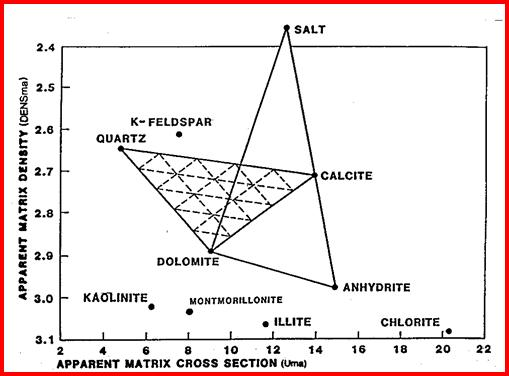Matrix Density – Matrix Cross Section Crossplot for LithologyLithology From Spectral Gamma Ray Data

Many other two and three mineral models can be made using combinations of sonic, density, neutron, PE and spectral gamma ray log curves, such as potassium or thorium or potassium/thorium ratio. Typical crossplots are shown on the following page.

A comparison of various lithology methods is shown on Figure PP6.19. Notice that some models could not resolve for three minerals so the anhydrite zone is not portrayed correctly.PE vs Th/K Ratio Crossplot for Lithology. X Axis Th/K (ppm/%)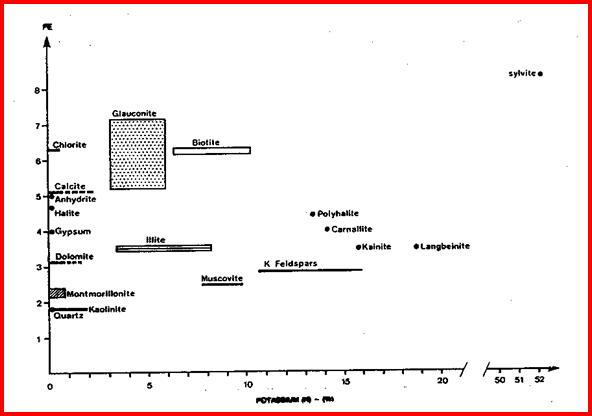PE vs Potassium Crossplot for Lithology.  X Axis is K (%).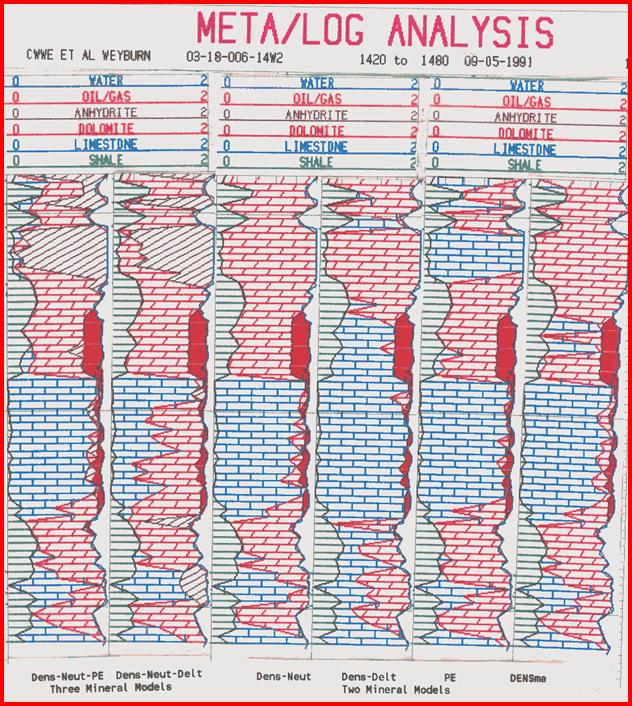Comparison of Lithology Methods. Note that the 2-mineral model will give silly results in a 3-mineral environment, as shown here in the anhydrite layer, unless appropriate parameters and zoning are applied.Lithology From Vp/Vs Velocity Ratios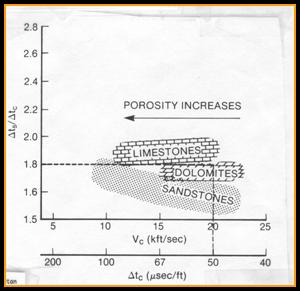The dipole shear sonic log provides compressional and shear travel time values that can be used for lithology estimation.

1: Vp/Vs = DTS  / DTC

Where DTC = compressional travel time (usec/ft or usec/m)

DTS = shear travel time (usec/ft or usec/m)

Vp/Vs = velocity ratio (unitless)

This ratio is used in seismic calibration and interpretation as a guide to lithology. Vp/Vs is relatively independent of porosity and fluid type (little gas effect).

RECOMMENDED PARAMETERS:

Sandstone Vp/Vs = 1.50 – 1.70

Dolostone Vp/Vs  = 1.65 – 1.80

Limestone Vp/Vs  = 1.80 – 2.00

Page Views ---- Since 01 Jan 2015